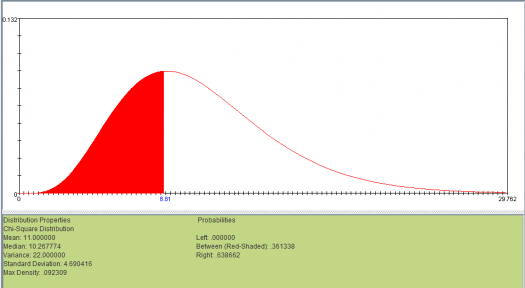# The Chi-square Tests Assessment Test

10 Questions | Total Attempts: 687SettingsTake our assessment test to assess your knowledge of the chi-square tests. The chi-square goodness of fit test that determines if a sample data matches a population and the chi-square test for independence that compares two variables in a contingency table to see if they are related.

• 1.
What is the null hypothesis in chi-square test?
• A.

The rows and columns are the same

• B.

The rows and columns in the table are associated

• C.

The rows and columns in the table are not associated

• D.

The rows and column are not the same

• 2.
The least expected value in each contingency table cell in order for a chi-square test to be effective is...
• A.

5

• B.

4

• C.

3

• D.

2

• 3.
Contingency tables and degrees of freedom are key elements in chi-square test?
• A.

False

• B.

Maybe

• C.

True

• D.

None

• 4.
What is another name for the chi-square goodness fit test?
• A.

Directional chi-square

• B.

One sample chi-square

• C.

Chi-square Anova

• D.

Two sample chi-square

• 5.
What type of data do you use for a chi-square table?
• A.

Ratios

• B.

Scales

• C.

Ordinal

• D.

Categorical

• 6.
How many cases must appear in one category of a chi-square test?
• A.

4

• B.

6

• C.

5

• D.

7

• 7.
What is an effective size?
• A.

The magnitude of the relationship between variables

• B.

The likelihood of type 1 and type 2 errors

• C.

The number of expected cases

• D.

The variance explained by the measures

• 8.
How can low expected values be dealt with?
• A.

Add more of the same number

• B.

Increase the sample size or combine categories

• C.

Exclude outliers

• D.

None of these

• 9.
By which other name is a chi-square contingency table analysis known?
• A.

Chi-square test for independence

• B.

Chi-square test for dependence

• C.

Chi-square variable test

• D.

None of the above

• 10.
Which of these symbols is used to represent a chi-square test?
• A.

C

• B.

Q

• C.

T

• D.

X2

Related TopicsBack to top